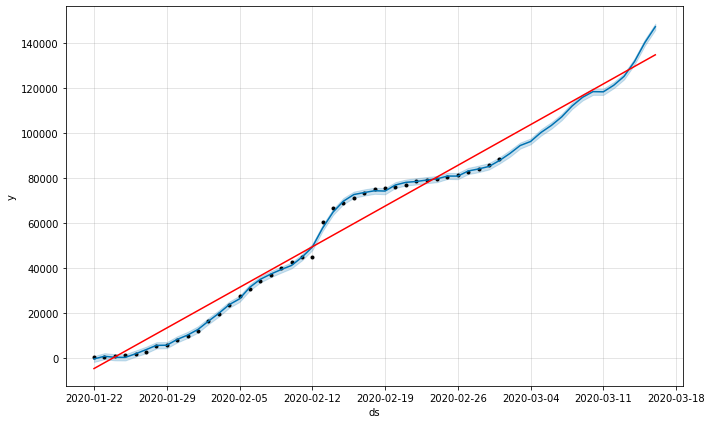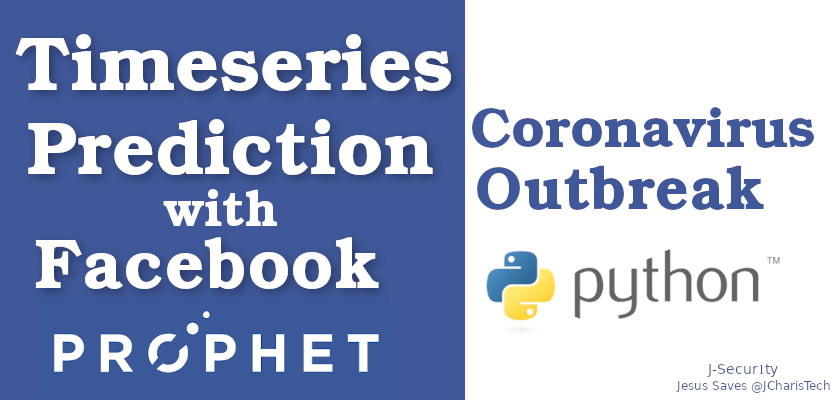# Predicting Coronavirus Outbreak with Facebook Prophets – A Timeseries Forecast

The recent increase in Coronavirus (Covid 19)  outbreak is gathering several concerns. In this tutorial we will try to see if we can make some predictions and time series forecasting using the data available now.

We will be using Facebook Prophet – an opensource package by Facebook for time series forecasting and prediction with pystan as a dependence.

But before we start, there are some basic things to take note of.

Less Data : Since the data available now is not that much our prediction may not be that accurate.

Presence of Effective Treatment or Vaccine or Medication: In the case of availability of an effective treatment or medication , the result of the outbreak will drastically decline which can affect our prediction.

Let us start. We will be using the dataset from John Hopkins on Github. Let us see the basic workflow for our task.

• Fetch and Prepare Data
• Group our Data by Dates
• Rename our Column specifically as ds and y for FB Prophet
• Split our Dataset into Train and Test
• Build our model and make prediction
• Plot predictions

Installation of FB Prophet

`pip install fbprophet`

We will be using a simple function to fetch and prepare our current data.

In :
```confirmed_cases_url = "https://raw.githubusercontent.com/CSSEGISandData/COVID-19/master/csse_covid_19_data/csse_covid_19_time_series/time_series_19-covid-Confirmed.csv"
recovered_cases_url ="https://raw.githubusercontent.com/CSSEGISandData/COVID-19/master/csse_covid_19_data/csse_covid_19_time_series/time_series_19-covid-Recovered.csv"
death_cases_url ="https://raw.githubusercontent.com/CSSEGISandData/COVID-19/master/csse_covid_19_data/csse_covid_19_time_series/time_series_19-covid-Deaths.csv"
```
In :
```def get_n_melt_data(data_url,case_type):
melted_df = df.melt(id_vars=['Province/State', 'Country/Region', 'Lat', 'Long'])
melted_df.rename(columns={"variable":"Date","value":case_type},inplace=True)
return melted_df

def merge_data(confirm_df,recovered_df,deaths_df):
new_df = confirm_df.join(recovered_df['Recovered']).join(deaths_df['Deaths'])
return new_df
```
In :
```# Load EDA pkg
import pandas as pd
```
In :
```confirm_df = get_n_melt_data(confirmed_cases_url,"Confirmed")
recovered_df = get_n_melt_data(recovered_cases_url,"Recovered")
deaths_df = get_n_melt_data(death_cases_url,"Deaths")
```

```df = merge_data(confirm_df,recovered_df,deaths_df)
```

After that we will group our data by dates and then rename the dates as ds and the confirmed cases/recovered cases as y. This is the accepted format for feeding data into fbprophet. Hence we have to rename them as such.

```df_per_day = df.groupby("Date")[['Confirmed','Recovered', 'Deaths']].sum()
```
`global_cases = df_per_day.reset_index()`
```confirmed_cases = global_cases[["Date","Confirmed"]]
recovered_cases = global_cases[["Date","Recovered"]]```
`confirmed_cases.rename(columns={"Date":"ds","Confirmed":"y"},inplace=True)`

Since we do not have enough data and we want to validate our prediction we will split our dataset into train and test dataset. You can ignore this step if you want but we want to compare our prediction using FB prophet with our test dataset.

Based on how much data we have we will split it into 80/20 and use the 20 as our test data.

```train = confirmed_cases[:40]
test = confirmed_cases[40:]
```

After preparing our data we will then feed it into our model and fit and train our model.

FB Prophet has some interesting parameters that you can adjust to fit your prediction and dataset but we will leave it at the default and add a monthly seasonality to it.

```# Model Initialize
from fbprophet import Prophet
m = Prophet()
```
In :
```m.add_seasonality(name="monthly",period=30.5,fourier_order=5)
```
```# Fit Model
m.fit(train)```

We will then use the make_future_dates function to generate 15 days and then use it for our prediction.

```# Future Date
future_dates = m.make_future_dataframe(periods=15)```
```# Prediction
prediction =  m.predict(future_dates)
```
In :
```# Plot Prediction
m.plot(prediction)
```
Out: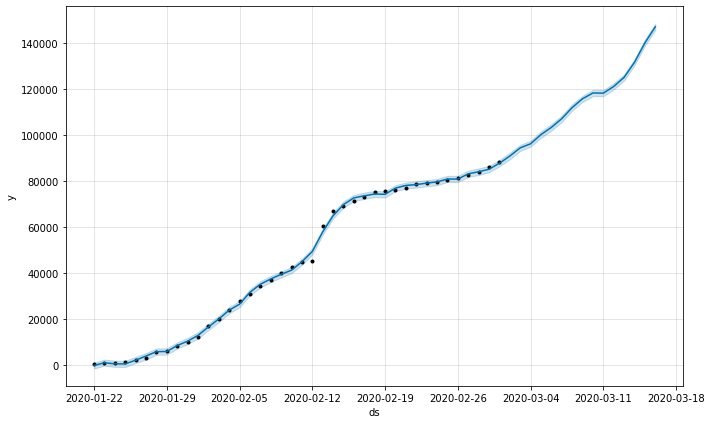In :
```m.plot_components(prediction)
```
Out: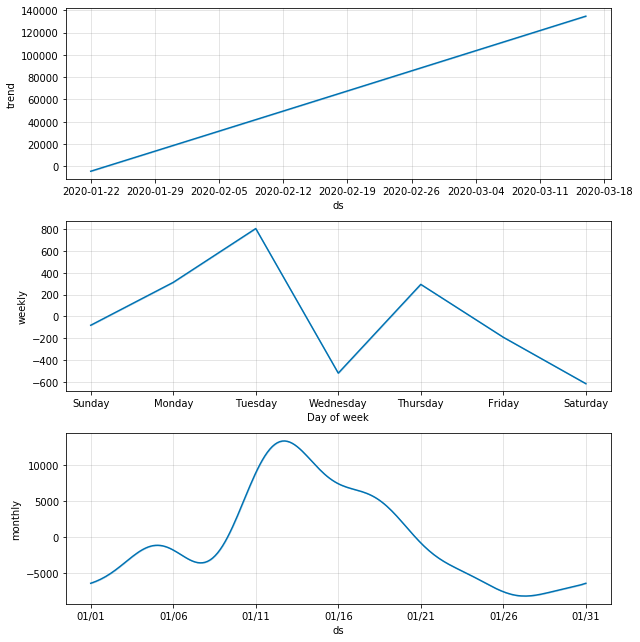`test['dates'] = pd.to_datetime(test['ds'])`
```test = test.set_index("dates")
```
In :
```test = test['y']
```
In :
```import matplotlib.pyplot as plt
```
In :
```test.plot()
```
Out:
`<matplotlib.axes._subplots.AxesSubplot at 0x7f83f6bcd048>`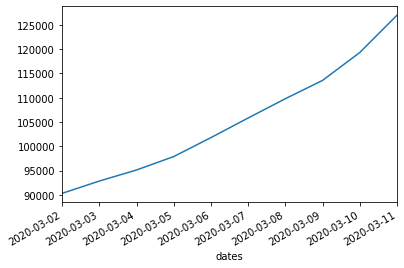Based on our prediction and our plot for the test dataset we can see some similarity in our plot. It looks like our model is on track as it shows an increase in the number of confirmed cases – a rising trend just like our test data set showsI
We can also use the add_changepoints_to_plot function to check the points in which there were changes in the trend.
:
```# Find Point/Dates For Change
```fig = m.plot(prediction)